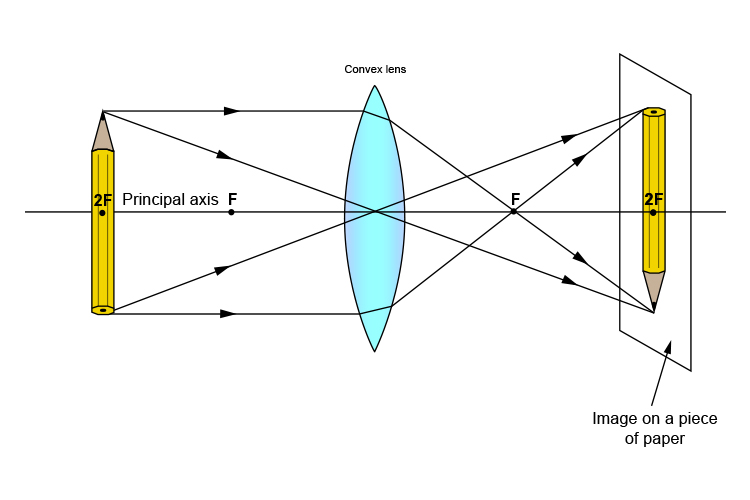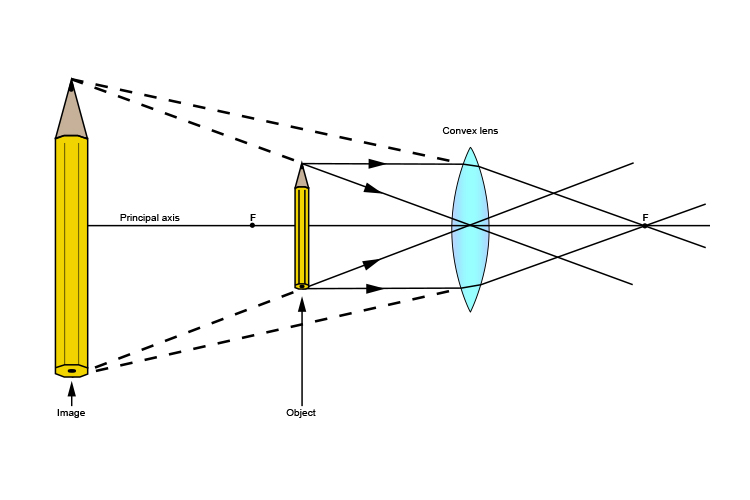# Object passes through principal axis

So far, in all our diagrams of convex lenses, we have only had an object on one half of the principal axis.

This is purely done to keep the learning process simple and in stages, but it is important to realise that each of the lessons works exactly the same for the other half of the principal axis.

If the object passes through the principal axis, the drawings get more complicated but all the ideas work exactly the same way. See below:The same ideas work either side of the principal axis.

This works for objects that straddle the principal axis at less than one principal focal length from the lens, too: